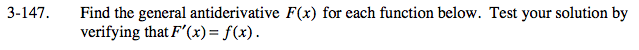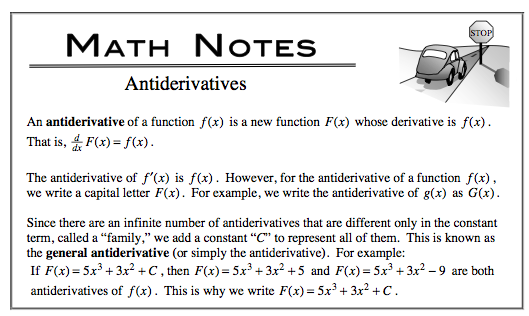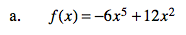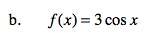### Home > CALC > Chapter 3 > Lesson 3.4.1 > Problem3-147

3-147.
1. Find the general antiderivative F(x) for each function below. Test your solution by verifying that F ′(x) = f(x). Homework Help ✎

1. f(x) = −6x5 + 12x2

2. f(x) = 3 cos xUndo the Product rule.

Do not forget the +C.Do not forget the +C.